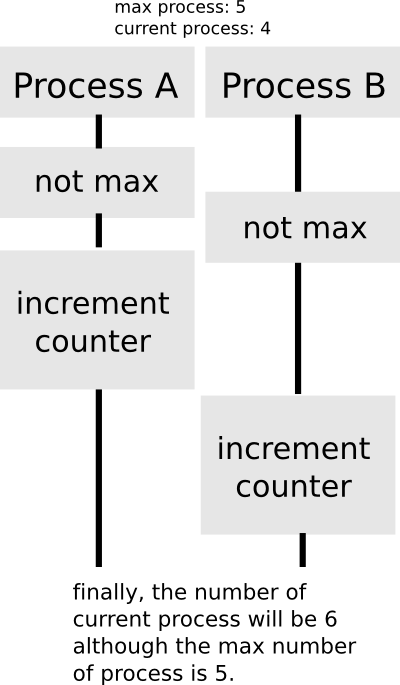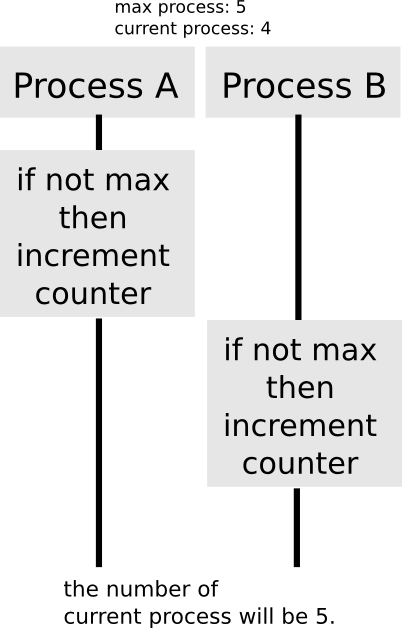## [SICP Excercise 3.47] Some observation about semaphore

7-14-2014

```(define (make-semaphore n)
(let ((the-mutex (make-mutex))
(count 0))
(define (the-semaphore m)
(cond ((eq? m 'acquire)
(the-mutex 'acquire)
(if (= count n)
(begin
(the-mutex 'release)
(the-semaphore 'acquire))
(begin
(set! count (+ count 1))
(the-mutex 'release))))
((eq? m 'release)
(the-mutex 'acquire)
(if (> count 0)
(set! count (- count 1)))
(the-mutex 'release))))
the-semaphore))
```

(I’ve Borrowed code above from Weiqun Zhang’s Blog – SICP Exercise 3.47.)

It took some time to understand the structure of semaphore. Here is the problem:

## The place of ‘acquire’

Next question that bothered me was that: does the code still work if I replace acquire method as follows?

```(define (make-semaphore n)
(let ((the-mutex (make-mutex))
(count 0))
(define (the-semaphore m)
(cond ((eq? m 'acquire)
(if (= count n)
(the-semaphore 'acquire))
(begin
(set! count (+ count 1))
(the-mutex 'release))))
((eq? m 'release)
(the-mutex 'acquire)
(if (> count 0)
(set! count (- count 1)))
(the-mutex 'release))))
the-semaphore))
```

After a little bit observation I noticed that this code has a problem:So the place of ‘acquire’ should be before (if (= count n) … :